# Bessel functions

(diff) ← Older revision | Latest revision (diff) | Newer revision → (diff)
Jump to: navigation, search

Cylinder functions of the first kind. A Bessel function of ordercan be defined as the series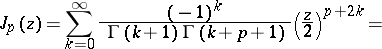(*)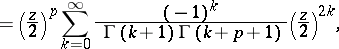which converges throughout the plane. A Bessel function of orderis the solution of the corresponding Bessel equation. If the argument and the orderare real numbers, the Bessel function is real, and its graph has the form of a damped vibration (Fig.); if the order is even, the Bessel function is even, if odd, it is odd.Figure: b015840a

Graphs of the functions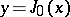and.

The behaviour of a Bessel function in a neighbourhood of zero is given by the first term of the series (*); for large, the asymptotic representation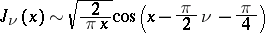holds. The zeros of a Bessel function (i.e. the roots of the equation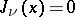) are simple, and the zeros of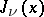are situated between the zeros of. Bessel functions of "half-integral" order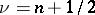are expressible by trigonometric functions; in particularThe Bessel functions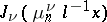(whereare the positive zeros of,) form an orthogonal system with weightin the interval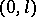. Under certain conditions the following expansion is valid: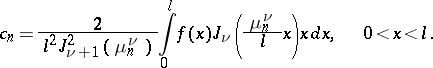In an infinite interval this expansion is replaced by the Fourier–Bessel integral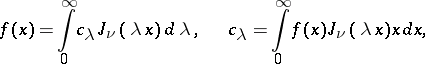The following formulas play an important role in the theory of Bessel functions and their applications:

1) the integral representation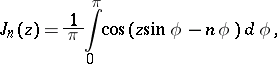2) the generating function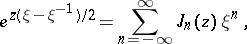3) the addition theorem for Bessel functions of order zero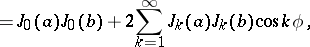4) the recurrence formulas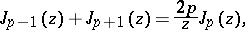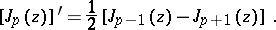For references, see Cylinder functions.

How to Cite This Entry:
Bessel functions. Encyclopedia of Mathematics. URL: http://encyclopediaofmath.org/index.php?title=Bessel_functions&oldid=14104
This article was adapted from an original article by P.I. Lizorkin (originator), which appeared in Encyclopedia of Mathematics - ISBN 1402006098. See original article